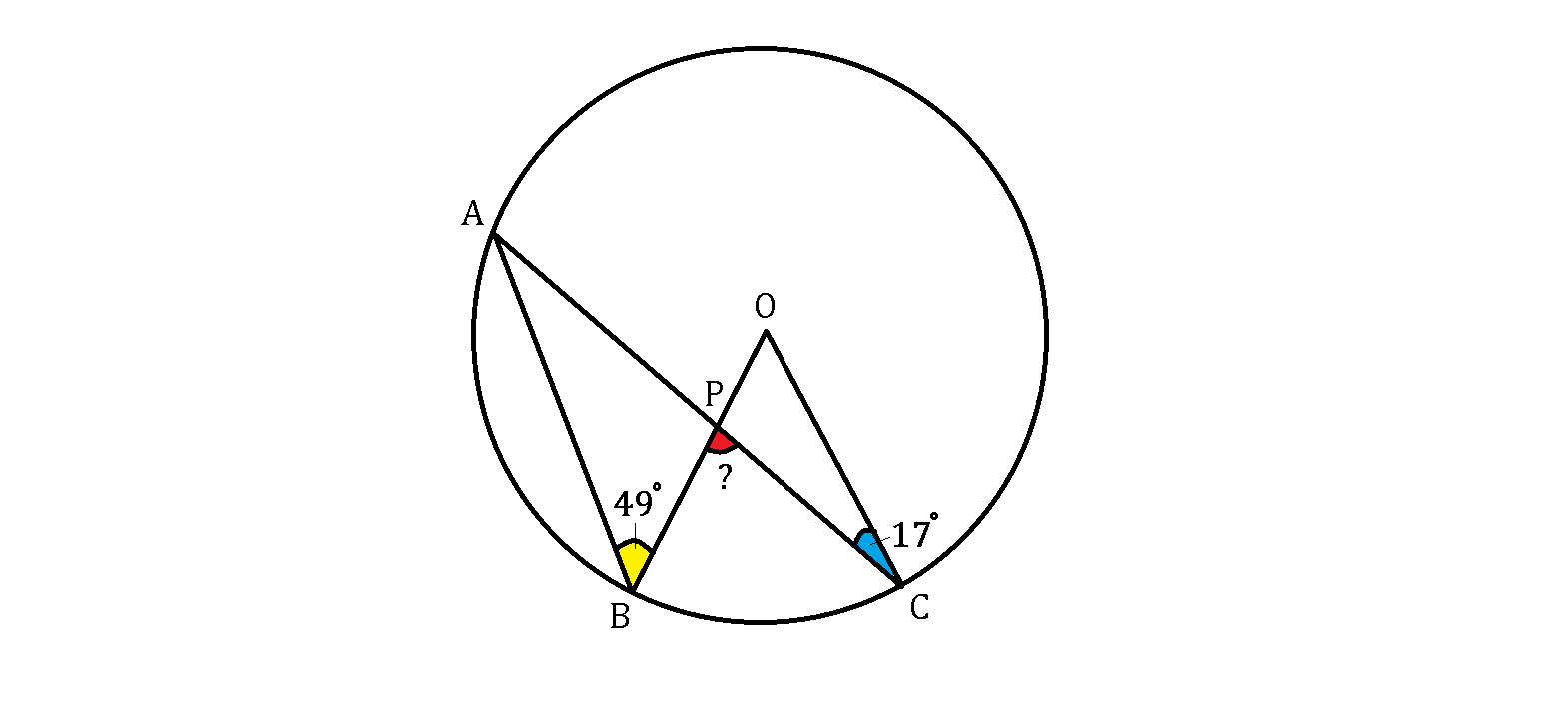# Off Center AngleThe points $A, B, C$ are on a circumference, where $O$ is the center, and $P$ is the intersection point of $AC$ and $BO$, as shown above.

If $\angle ABP = 49^{\circ}$ and $\angle PCO = 17^{\circ}$, what is the value of $\angle BPC$ in degrees?

×